# What is Ohms Law?

### What is Ohms Law

Ohm’s Law is one of the most fundamental of laws in electrical theory. If you open an electrical basic book, you will see in the first which is named as “Ohm’s Law”. The Ohm’s law is derived by German physicist George Simon Ohm. The Ohms Law links voltage, current, and resistance of the conductor in a circuit. Ohm’s Law is one of the most fundamental and important laws governing electrical and electronic circuits.

### History of Ohm’s Law

There is a mathematical relationship which links current, voltage and resistance. A German scientist named Georg Ohm performed many experiments in an effort to show a link between the three. In the days when he was performing his experiments, there were no meters as we know them today.

Only after considerable effort and at the second attempt did he manage to devise what we know today as Ohm’s Law.

### Ohm’s Law definition

Ohm’s Law states that at a constant temperature or the physical property remains constant, the voltage across a resistor is directly proportional to the current flowing through the resistor or conductor.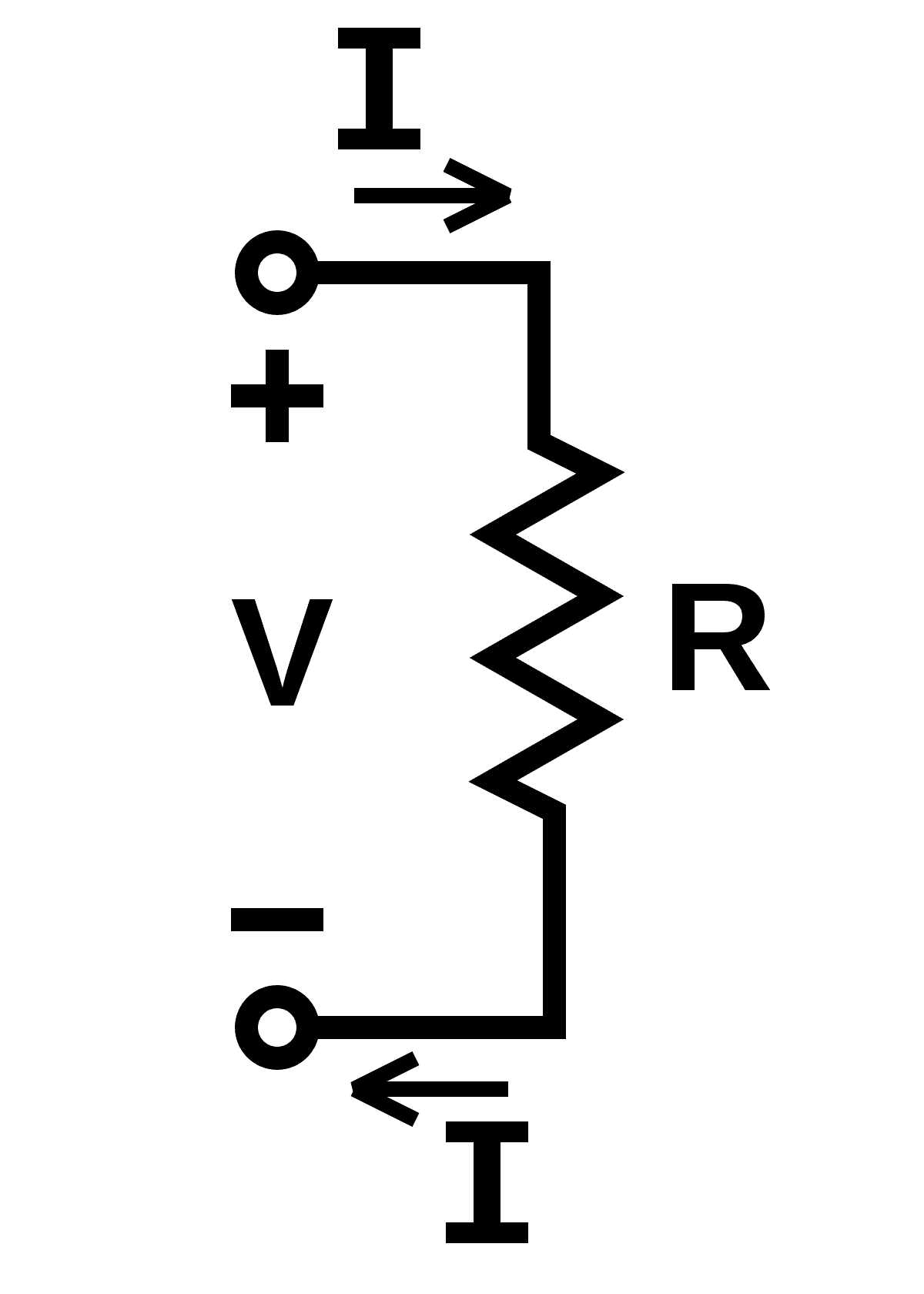Let us consider voltage or potential difference V across the terminal, I is the current flowing through the conductor, the applied voltage V is directly proportional to Current I, let’s make it simple…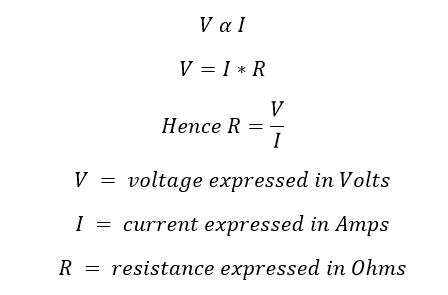The proportionality constant R is the resistance of the conductor and the unit of resistance is measured in Ohms.

In other words, by doubling the voltage across a circuit the current will also double. However, if the resistance is doubled the current will fall by half.

Example: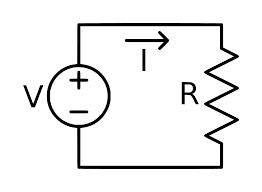### Definition for 1 Ohm:

A conductor said to be one ohm if the circuit permits one-ampere current for one volt applied across the circuit terminal.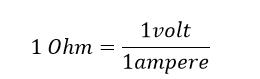If 1 ampere of current is flowing through a resistance, and 1 Watt/hour energy (dissipate in the form of heat) is generated, then the measurement of that resistance is 1 Ω

## Ohm’s law triangle (How to remember)

Are you a student, this ohm’s law triangle may help you to remember Ohm’s law easily? This is sometimes known as the Ohm’s law triangle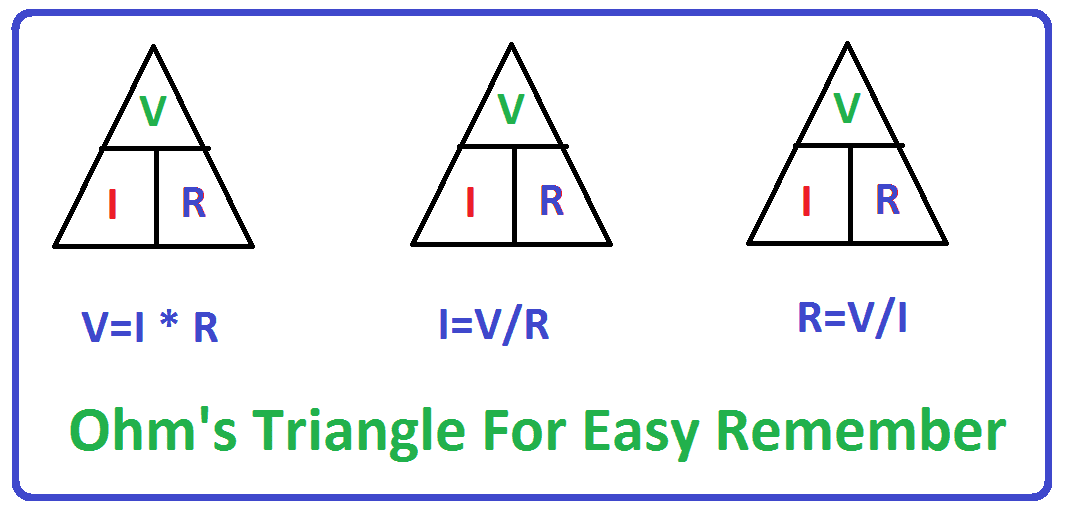In the top corner of the Ohms law triangle is the letter V is the Voltage or the potential difference, in the left-hand corner of the triangle, the letter I, and in the right-hand bottom corner letter, R is the resistance of the circuit.

### How to use the triangle:?

To use the triangle, cover up the unknown quantity and then and then calculate it from the other two. If they are in line they are multiplied, but if one is on top of the other then they should be divided.

For example: if you want to know the current flowing in the circuit means, the top of the triangle letter V voltage is divided by the left corner of the letter R. Remaining thing and so on.

But in Some case such as The Voltage V of the circuit has to be calculated, then you should multiply the current I by the resistance R times.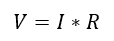Best example: if a voltage of 20 volts is applied across a circuit which has a 1000-ohm resistor, calculate the current flowing through the circuit. Looking at the ohm’s triangle the current is the unknown leaving the voltage and resistance as the known values. The current is calculated by dividing the voltage by the resistance.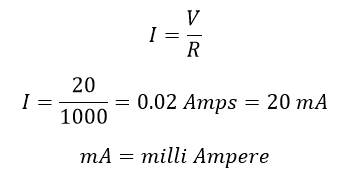## Linear graph

The graph shows the relationship between the voltage, current and resistance of the circuit. It can be seen that if the voltage and current were plotted for a fixed resistor or a length of wire, etc, there would be a linear curve, on constant temperature.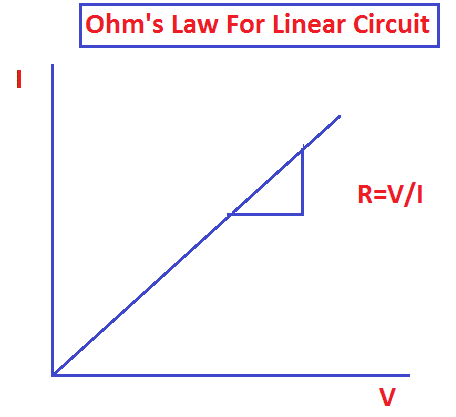Plot of voltage and current for a linear resistance

The slope of the graph gives the resistance of the circuit. Here the low resistance shows a component that requires a lower voltage to be applied for a given current. one the other hand for a higher resistance – this one requires more voltage to be applied for a given current flowing. Accordingly, the circuit must have a higher resistance.

## Limitation on Ohm’s law:

1. Ohm’s law cannot be used when the temperature of the circuit keeps on changing, it is applicable to constant temperature’s circuit.
2. It cannot apply to non-linear components such as semiconductor circuit.

## Ohm’s law application:

It is used to find out the circuit’s unknown quantity such as voltage or current or resistance. All electrical student must know about ohm’s law concept.